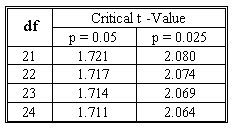### CFA Practice Question

There are 208 practice questions for this study session.

### CFA Practice Question

Beth has two independent variables and 25 observations in her regression analysis. The t-statistic for one of the independent variables X1 is 1.83. She wants to test H0: β1 <= 0 against H1: β1 > 0. What is the critical value for Student's t distribution and is the variable significant at the 5% confidence level?A. The critical value is 1.714, and the variable is significant.
B. The critical value is 1.717, and the variable is significant.
C. The critical value is 2.060 and the variable is not significant.
Explanation: The degrees of freedom: 25 - 2 - 1 = 22.
Critical value t0.05, 22: 1.717.
As 1.717 < 1.83, the variable is significant at the 5% confidence level.